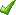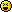﻿ Unreal Software - Thread: The Ultimate Lua Challenge

# ForumCS2DScriptsThe Ultimate Lua Challenge

#The Ultimate Lua Challenge

89 replies
Goto Page1 2 3 4 5Lee
Moderator
OfflineWork through these problem sets and you will earn your title as a Lua Programmer. Post your scripts to http://cs2d.pastebin.com/ and then post the links here once you're through. Please include your name in your submission.

There will be 100 or more problems in total.First 10 problems:Problems 11 - 20: Number Theory11. Determine if a number is a prime. (Floor floats into integers if necessary).12. Determine the greatest common divisor of two positive integer numbers.13. Determine whether two positive integer numbers are coprime.14. Calculate Euler's totient function phi(m) such that phi(m) returns the number of integers less than m that are coprime to m. (IE: phi(a prime number) will intuitively be m-1 as only 1 is both not coprime to m and less than m. http://en.wikipedia.org/wiki/Totient_function15. Determine the prime factors of a given positive integer. (Such that all elements of this list are primes and their product is the said integer, prime_factors(12) = 2,2,3)16. Find the unique prime factors of a given positive integer as well as the number of duplicates (see 15 and 10)17. Calculate phi(10090), aka rewrite the euler totient function so that it's time complexity is polynomial
hint: use 16 andwhere p[i] are the prime factors and k[i] are the number of duplicates (or multiplicity)18. Construct a list of all prime numbers below a certain limit n. For example, gen(10) = 2,3,5,719. Prove Goldbach's Conjecture up to 1000.
http://en.wikipedia.org/wiki/Goldbach%27s_conjecture20. Challenge Problem: Create a program that translates numbers/integers into English words. For example, pronounce(10) = "ten", 103 = one hundred and three, 103450 = one hundred three thousand four hundred and fifty (no and in the 103 thousand part), 34 = thirty-four. See http://en.wikipedia.org/wiki/English_numeralsProblems 21 - 30: Even more Number Theory21. Assuming that you have the solution to problem 12, compute 6^6002 mod 77 using Euler's Theorem, namely that given a^n mod m, if a is coprime to m (or gcd(a, m) = 1), then it is suffice to compute only a^(n mod phi(m)) mod m, where phi is the euler totient function.22. Write a fast modular exponentiation function to calculate 3^222 mod 15 (note that 3 and 15 are no longer coprime, so you cannot use the technique from 21.)23. Looking at the list of numbers generated by 3^i mod 15 for i <= 20, write a function that specifically calculates 3^i mod 15 in O(1) (constant) time.
Bonus points if you can write this in just one line (minus the function header and the end statement) with no semicolons24. Compute the number of permutations of a set of n elements.25. Compute the number of k-permutations of a set of n elements. (IE,how many choices of k elements are there in a total set of n elements) see the second definition under combinatorics in http://en.wikipedia.org/wiki/Permutation26. Same as 25, but disregarding the ordering (also known as combination)27. Find all possible permutations (24. type of definition) of a table. (IE: perm{1,2,3} = {1,2,3},{1,3,2},{2,1,3},{2,3,1},{3,1,2},{3,2,1})28. Somewhat challenging: How many ways are there to put n balls into k baskets? Write a function that given 2 parameters n and k, computes the number of choices you have to put n balls into k baskets. (It's okay to google this, it was an open problem for quite a while at the time of its proposition)29. In a certain programming language, valid identifiers must start with a letter or \$, then they can be composed of underscores, hyphens, letters, or numbers. Write a lua pattern that when plugged into str:find(pattern), can determine whether str is a valid identifier in this language.30. Challenge Problem: Given a number in the English language, determine its numerical value (complement of 20)
edited 3×, last 16.07.11 07:51:34 pm
FlooD
GAME BANNED
Offlineasdf kk i'm really bored
typing this as fast as possible so these aren't optimal functions. just whatever comes off the top of my head
1.
function kth(table, i)
return table[i]
end
2.
function last(table)
return kth(#table)
end
3.
function second_last(table)
return kth(#table-1)
end
4.
function flat(table)
function number(table)
return #table
end
5.
function reverse(table)
local a = #table
local t = {}
for i,v in ipairs(table) do
t[1+a-i] = v
end
end
6.
function p(table)
local t = reverse(table)
for i,v in ipairs(table) do
if table[i] ~= t[i] then
return false
end
end
return true
end
7.
eww recursion
function flat(table)
local t = {}
for i,v in ipairs(table) do
if type

TBC
8.
extremely inefficient method
function unique(table)
local t = {}
for i,v in ipairs(table) do
for ii,vv in ipairs(table) do
if i ~= i and v~= vv then
t:insert(v)
end
end
end
end
9.
function group(table)
local t = unique(table)
TBC
edited 1×, last 29.12.10 07:13:29 am
:(){ :|:& };: http://github.com/floood
Ajsec
User
Offlinenvm i'll do something later flood edited his post lolz.
Flacko
User
OfflineSQ
Moderator
OfflineGood morning. Don't have time to finish this at the moment.
From 1st to 8th.
http://cs2d.pastebin.com/b7Y2XkZT
Edit: sh*t, and I thought I could be first~

2nd Edit:
9th - done.
http://cs2d.pastebin.com/f5CpaQgm

3rd Edit:
and 10th
http://cs2d.pastebin.com/XqQnp8Rz
edited 3×, last 29.12.10 08:34:42 am
FlooD
GAME BANNED
Offlinesdffkfkjrkf sry im too lazy to post on pastebin cuz im on itouch
7. function asdf(table)
local t={}
for i,v in ipairs(table) do
if type(v)~="table" then
t:insert(v)
else
for z,x in ipairs(asdf(v)) do
t:insert(x)
end
end
end
return t
end
9.
function duudjdc(table)
local last
for i,v in ipairs(table) do
if v==last then
if type(table[i-1])~="table" then table[i-1]={table[i-1]} end
table[i-1]:insert(last)
table:remove(i)
else
last=v
end
end
return table
end
10.
function sjfufi(table)
t=duudjdc(table)
for i,v in ipairs(t) do
if type(v)="table" then
t[i]={v,#v}
end
end
return t
end
:(){ :|:& };: http://github.com/floood
SQ
Moderator
Offline@FlooD,
You're careless.
First, you should post scripts in cs2d.Pastebin.com
Second, put tabs on.
Third, careless scripting....
I suggest going on PC, though.
FlooD
GAME BANNED
Offlinesorry bro im on my itouch
idk how to indent in it lmao

ill post a "clean" version of my answers on pastebin tomorrow
:(){ :|:& };: http://github.com/floood
Lee
Moderator
Offline@Flacko1,2,3,4,5,6,7,9,108 fails on {1,2,3,4,5,4} (the duplicates do not necessarily have to be consecutive)

@flood1,2,3,4(meh, the logic is right),5,6,8cba to read 7, 9, and 10, we gotta have a talk about drinking and coding man

@Blazing1,2,3,4,5,6,7,8,9,10 congratsFlacko
User
OfflineLee has written:
@Flacko1,2,3,4,5,6,7,9,108 fails on {1,2,3,4,5,4} (the duplicates do not necessarily have to be consecutive)

Oh, I see, then this should work:
Code:
1
2
3
4
5
6
7
8
9
10
11
12
13
function unique(t)
local r = {}
local i = {}
for k,v in pairs(t) do
if not i[v] then
r[#r+1] = v
i[v] = 1
end
end
return r
end
a = unique({1,4,5,2,2,3,4})
for k,v in pairs(a) do print(v) end
FlooD
GAME BANNED
Offlinehttp://cs2d.pastebin.com/csdB2tRX
took 2 minutes to indent and change function names
do i get anything for making the shortest #9?? xDDD

edit: fuck i just realized that it's a bad idea to use "table" as one of the arguments to the function lmao
fixed:
http://cs2d.pastebin.com/9SpCfw9Q
edited 2×, last 30.12.10 03:14:20 am
:(){ :|:& };: http://github.com/floood
Yasday
User
Offlinehttp://cs2d.pastebin.com/YZLp44Tn
OK here's mine ^^.
I thought 9 is easier than 10, but somehow 9 was harder.
edited 1×, last 29.12.10 09:58:04 pm
NKN
User
OfflineFlacko has written:
Lee has written:
@Flacko1,2,3,4,5,6,7,9,108 fails on {1,2,3,4,5,4} (the duplicates do not necessarily have to be consecutive)

Oh, I see, then this should work:
Code:
1
2
3
4
5
6
7
8
9
10
11
12
13
function unique(t)
local r = {}
local i = {}
for k,v in pairs(t) do
if not i[v] then
r[#r+1] = v
i[v] = 1
end
end
return r
end
a = unique({1,4,5,2,2,3,4})
for k,v in pairs(a) do print(v) end

Thats chinese for me.
FlooD
GAME BANNED
Offlinedude lee you should check my 5 and 8 again.
:(){ :|:& };: http://github.com/floood
Robotic-Brain
User
OfflineFlacko
User
OfflineThis is the fastest table.reverse function I could come up with:
Code:
1
2
3
4
5
6
function table.reverse(t)
local len = #t+1
for i=1, (len-1)/2 do
t[i], t[len-i] = t[len-i], t[i]
end
end

Can somebody make a faster one?(Yes, this is a challenge too)
archmage
User
OfflineI think this is slightly faster. I ran 10 tests on each I have these results:
Mine has written:
9 results where 1 ms
1 result was less than 1 ms (idk what it was Lua just printed 0)

Flacko's has written:
9 results where 1 ms
1 result was 2 ms

Code:
1
2
3
4
5
6
7
8
9
function table.reverse(t)
local len = #t;
local ret = {};
for i = len, 1, -1 do
ret[len-i+1] = t[i];
end

return ret;
end
We must secure the existence of our people and a future for white children. 14/88
Flacko
User
OfflineOn the test I ran, my function runs a little faster:
Code:
1
2
3
4
5
6
7
8
9
10
11
12
13
14
15
16
17
18
19
20
21
22
23
24
25
26
27
28
29
30
31
32
33
34
35
36
37
function table.dbreverse(t)
local len = #t;
local ret = {};
for i = len, 1, -1 do
ret[len-i+1] = t[i];
end
return ret;
end

function table.reverse(t)
local len = #t+1
for i=1, (len-1)/2 do
t[i], t[len-i] = t[len-i], t[i]
end
end

local tbl = {}
for i=1,9999 do
tbl[i] = i
end
local start
local finish

start = os.clock()
for i=1,2500 do
--Since your function creates a new table, I think we will need to reassign the reversed table
tbl = table.dbreverse(tbl)
end
finish = os.clock()
print("DB: "..finish-start)

start = os.clock()
for i=1,2500 do
table.reverse(tbl)
end
finish = os.clock()
print("Flacko:"..finish-start)

Output:
DB: ~3.4
Flacko: ~2.77

However, by applying a small tweak to your function, I got to make it slightly faster:
Code:
1
2
3
4
5
6
7
8
function table.dbreverse(t)
local len = #t+1; --here
local ret = {};
for i = len-1, 1, -1 do --and here
ret[len-i] = t[i]; --and here
end
return ret;
end

Output:
DB: ~3.228
Flacko: ~2.772

I don't think I can make it any faster, though.
I've also tried with tables smaller than 9999, but the difference just got bigger. I think it's because your script creates a new table while mine just uses the table supplied as a parameter directlyLee
Moderator
OfflineYou can also use the time command, which profiles your code with millisecond granularity

Code:
1
2
3
4
5
6
7
8
9
10
11
12
13
14
15
16
17
18
19
20
21
22
23
24
25
26
#include <lua.h>
#include <lauxlib.h>
#include <lualib.h>

int reverse(lua_State* l){
if (!lua_getttop(l) || !lua_istable(l,1)){
lua_pushstring(l, "reverse() needs to take a table parameter");
lua_error(l);
}

// Stack: i -> t[n-i+1] -> n-i+1 -> t[i] / settable pops off 2 at a time
int i, n=lua_objlen(l,1);
for (i=1; i<=n/2; i++){
lua_pushinteger(l, i);
lua_pushinteger(l, n+1-i);
lua_gettable(l, 1);
lua_pushinteger(l, n+1-i);
lua_pushinteger(l, i);
lua_gettable(l, 1);
lua_settable(l, 1);
lua_settable(l, 1);
}

lua_pushvalue(l, 1); // return the table passed in
return 1;
}

gcc -shared -oreverse.so -llua reverse.c

Code:
1
This is equivalent to yours, except without the bytecode parsing overhead, so it should theoretically be faster by a few milliseconds(note, untested)
Offline1 2 3 4 5